Anzeige

# 3. Venn Diagram.pptx

29. Mar 2023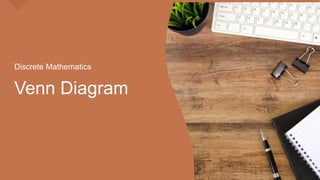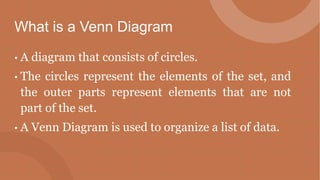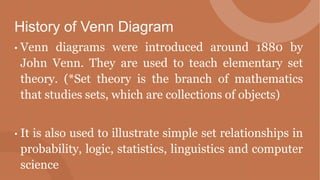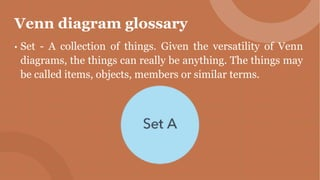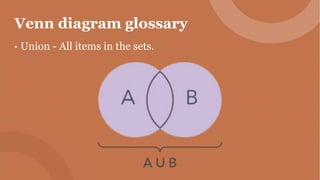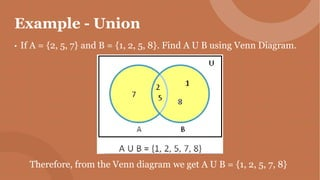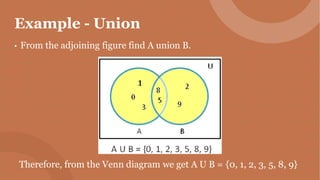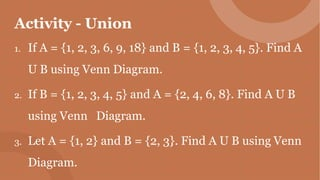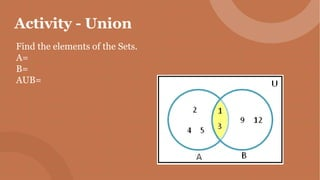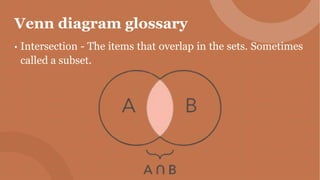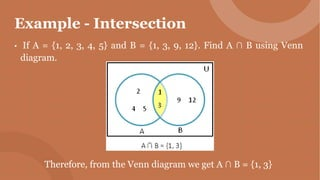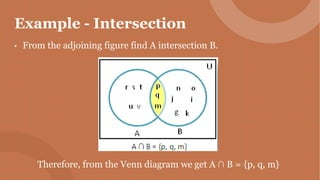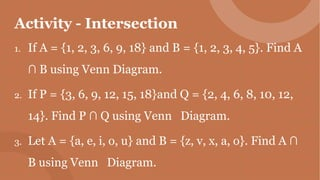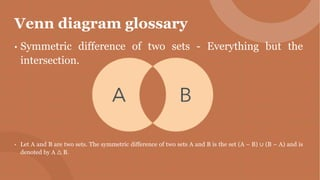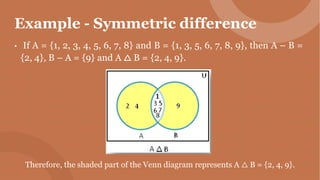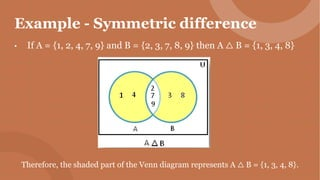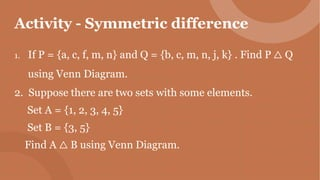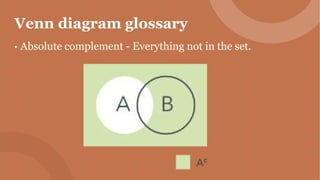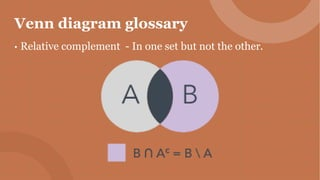1 von 21
Anzeige

### 3. Venn Diagram.pptx

1. Venn Diagram Discrete Mathematics
2. What is a Venn Diagram • A diagram that consists of circles. • The circles represent the elements of the set, and the outer parts represent elements that are not part of the set. • A Venn Diagram is used to organize a list of data.
3. History of Venn Diagram • Venn diagrams were introduced around 1880 by John Venn. They are used to teach elementary set theory. (*Set theory is the branch of mathematics that studies sets, which are collections of objects) • It is also used to illustrate simple set relationships in probability, logic, statistics, linguistics and computer science
4. History of Venn Diagram • Venn diagrams were introduced around 1880 by John Venn. They are used to teach elementary set theory. (*Set theory is the branch of mathematics that studies sets, which are collections of objects) • It is also used to illustrate simple set relationships in probability, logic, statistics, linguistics and computer science
5. Venn diagram glossary • Set - A collection of things. Given the versatility of Venn diagrams, the things can really be anything. The things may be called items, objects, members or similar terms.
6. Venn diagram glossary • Union - All items in the sets.
7. Example - Union • If A = {2, 5, 7} and B = {1, 2, 5, 8}. Find A U B using Venn Diagram. Therefore, from the Venn diagram we get A U B = {1, 2, 5, 7, 8}
8. Example - Union • From the adjoining figure find A union B. Therefore, from the Venn diagram we get A U B = {0, 1, 2, 3, 5, 8, 9}
9. Activity - Union 1. If A = {1, 2, 3, 6, 9, 18} and B = {1, 2, 3, 4, 5}. Find A U B using Venn Diagram. 2. If B = {1, 2, 3, 4, 5} and A = {2, 4, 6, 8}. Find A U B using Venn Diagram. 3. Let A = {1, 2} and B = {2, 3}. Find A U B using Venn Diagram.
10. Activity - Union Find the elements of the Sets. A= B= AUB=
11. Venn diagram glossary • Intersection - The items that overlap in the sets. Sometimes called a subset.
12. Example - Intersection • If A = {1, 2, 3, 4, 5} and B = {1, 3, 9, 12}. Find A ∩ B using Venn diagram. Therefore, from the Venn diagram we get A ∩ B = {1, 3}
13. Example - Intersection • From the adjoining figure find A intersection B. Therefore, from the Venn diagram we get A ∩ B = {p, q, m}
14. Activity - Intersection 1. If A = {1, 2, 3, 6, 9, 18} and B = {1, 2, 3, 4, 5}. Find A ∩ B using Venn Diagram. 2. If P = {3, 6, 9, 12, 15, 18}and Q = {2, 4, 6, 8, 10, 12, 14}. Find P ∩ Q using Venn Diagram. 3. Let A = {a, e, i, o, u} and B = {z, v, x, a, o}. Find A ∩ B using Venn Diagram.
15. Venn diagram glossary • Symmetric difference of two sets - Everything but the intersection. • Let A and B are two sets. The symmetric difference of two sets A and B is the set (A – B) ∪ (B – A) and is denoted by A △ B.
16. Example - Symmetric difference • If A = {1, 2, 3, 4, 5, 6, 7, 8} and B = {1, 3, 5, 6, 7, 8, 9}, then A – B = {2, 4}, B – A = {9} and A △ B = {2, 4, 9}. Therefore, the shaded part of the Venn diagram represents A △ B = {2, 4, 9}.
17. Example - Symmetric difference • If A = {1, 2, 4, 7, 9} and B = {2, 3, 7, 8, 9} then A △ B = {1, 3, 4, 8} Therefore, the shaded part of the Venn diagram represents A △ B = {1, 3, 4, 8}.
18. Activity - Symmetric difference 1. If P = {a, c, f, m, n} and Q = {b, c, m, n, j, k} . Find P △ Q using Venn Diagram. 2. Suppose there are two sets with some elements. Set A = {1, 2, 3, 4, 5} Set B = {3, 5} Find A △ B using Venn Diagram.
19. Venn diagram glossary • Absolute complement - Everything not in the set.
20. Venn diagram glossary • Relative complement - In one set but not the other.
21. Venn diagram glossary • Relative complement - In one set but not the other.
Anzeige